Chapter 13 Class 10 Surface Areas and Volumes

Class 10
Important Questions for Exam - Class 10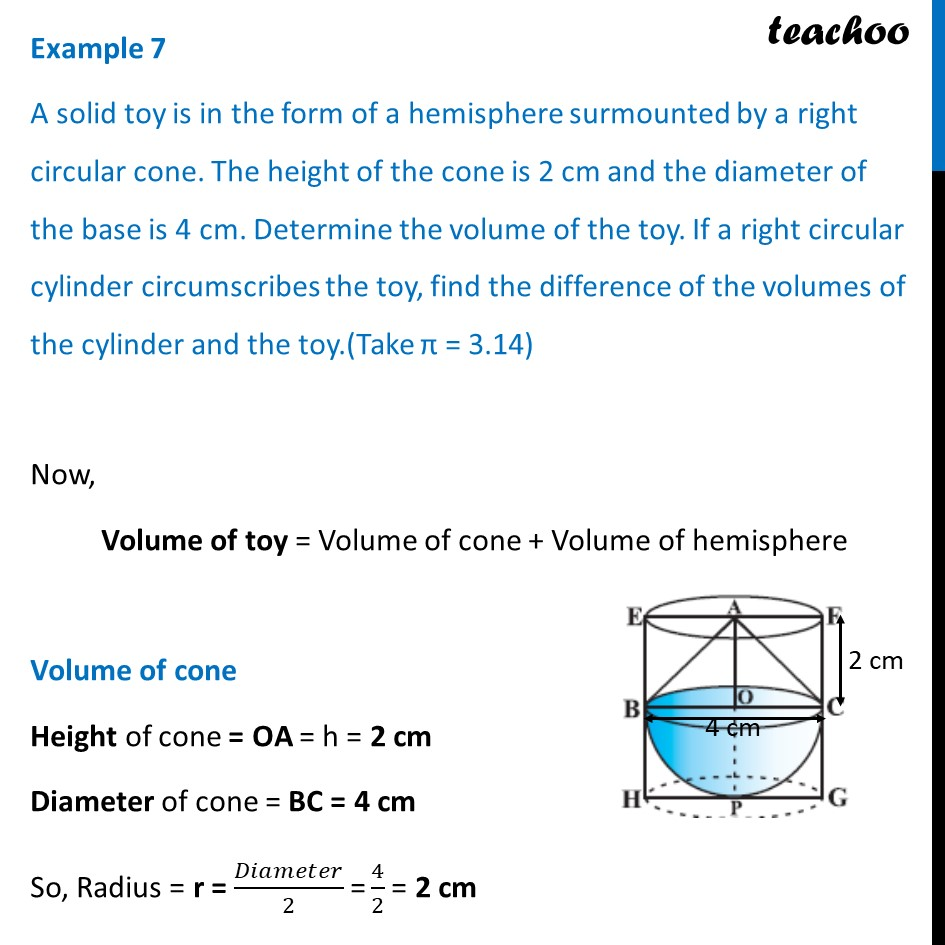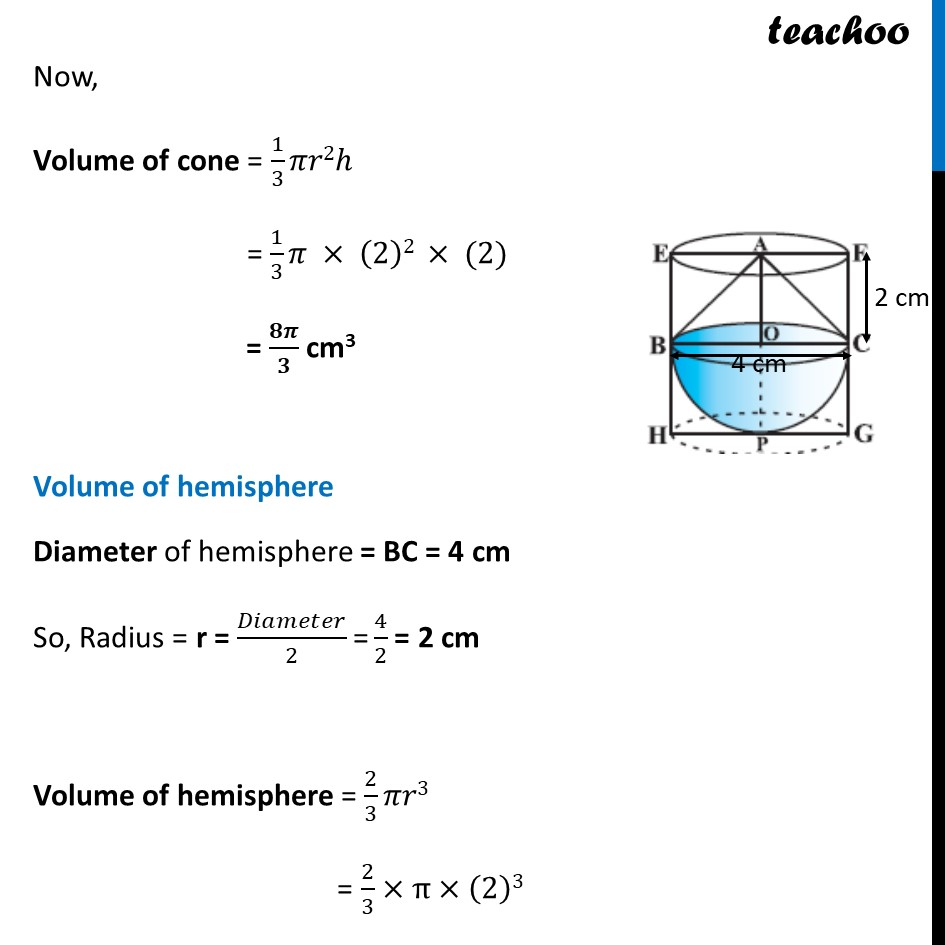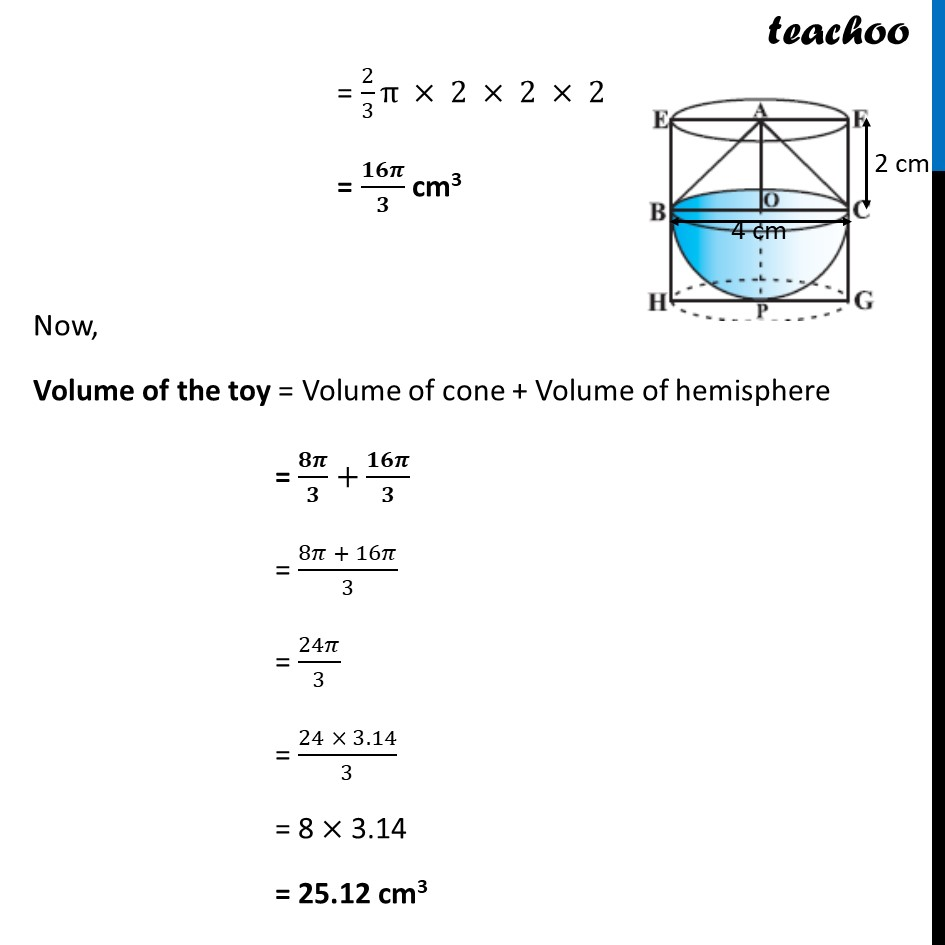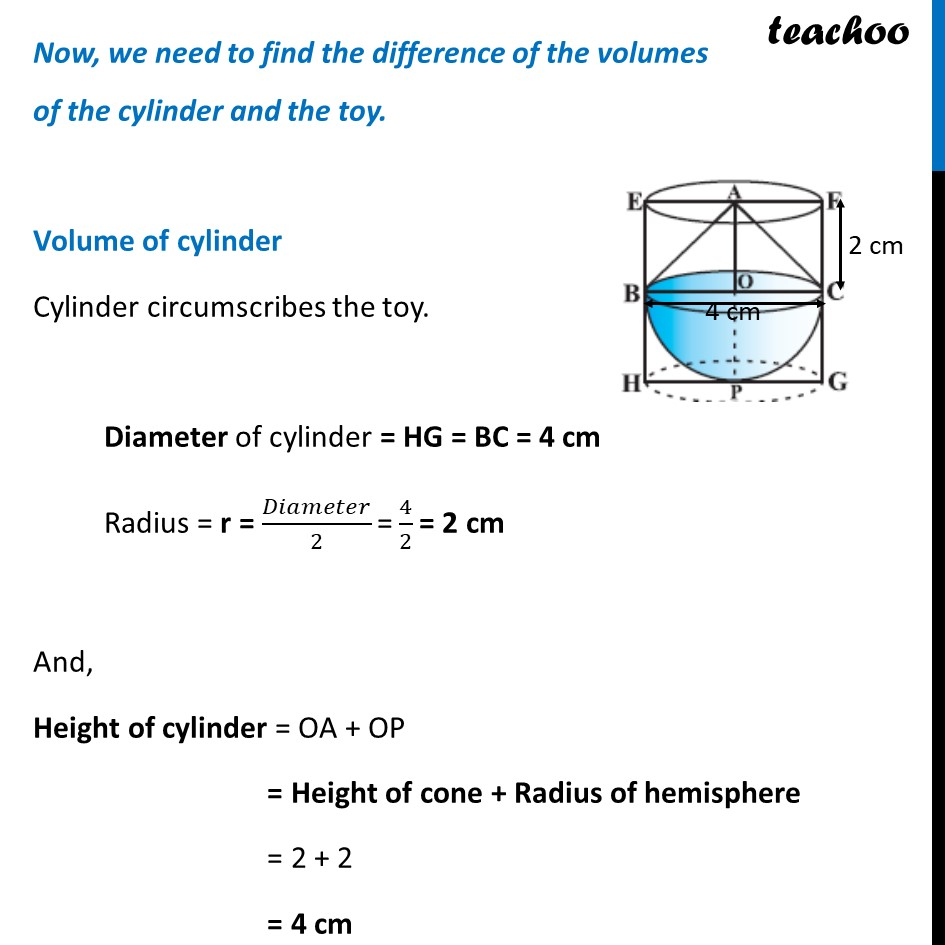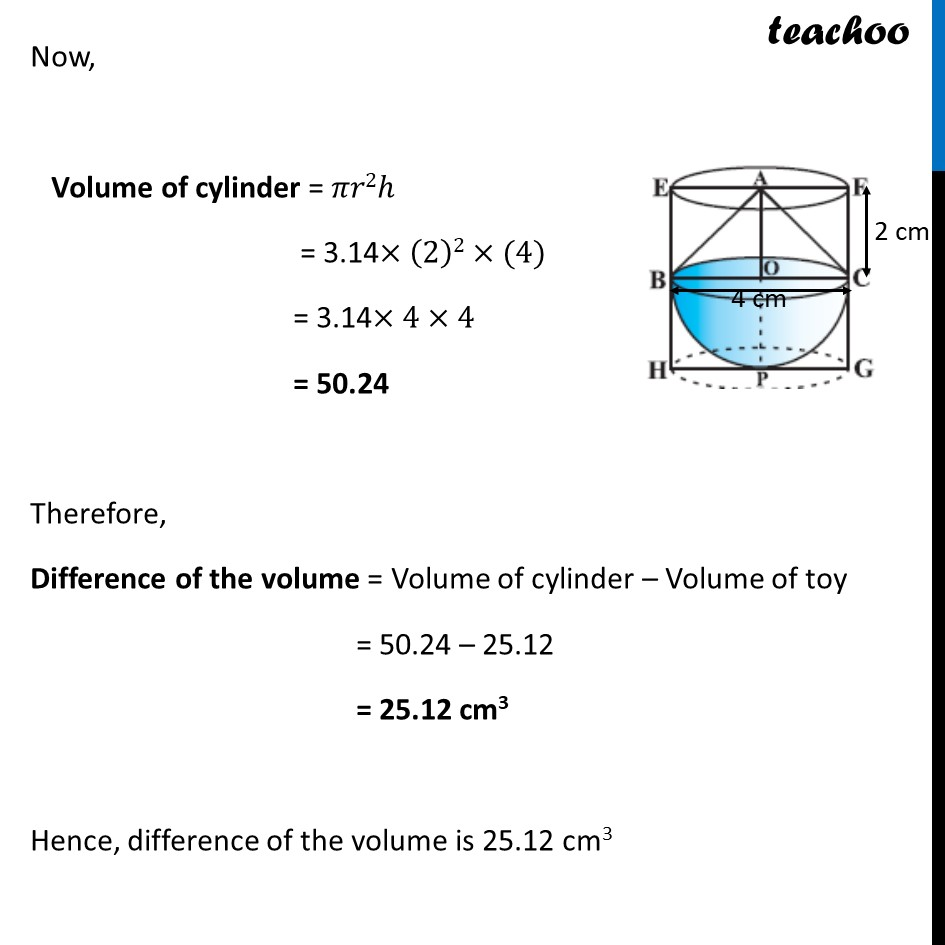Learn in your speed, with individual attention - Teachoo Maths 1-on-1 Class

### Transcript

Example 7 A solid toy is in the form of a hemisphere surmounted by a right circular cone. The height of the cone is 2 cm and the diameter of the base is 4 cm. Determine the volume of the toy. If a right circular cylinder circumscribes the toy, find the difference of the volumes of the cylinder and the toy.(Take π = 3.14) Now, Volume of toy = Volume of cone + Volume of hemisphere Volume of cone Height of cone = OA = h = 2 cm Diameter of cone = BC = 4 cm So, Radius = r = 𝐷𝑖𝑎𝑚𝑒𝑡𝑒𝑟/2 "=" 4/2 = 2 cm Now, Volume of cone = 1/3 𝜋𝑟2ℎ = 1/3 𝜋 × (2)2 × (2) = 𝟖𝝅/𝟑 cm3 Volume of hemisphere Diameter of hemisphere = BC = 4 cm So, Radius = r = 𝐷𝑖𝑎𝑚𝑒𝑡𝑒𝑟/2 "=" 4/2 = 2 cm Volume of hemisphere = 2/3 𝜋𝑟3 = 2/3×π×(2)3 = 2/3 π×2×2×2 = 𝟏𝟔𝝅/𝟑 cm3 Now, Volume of the toy = Volume of cone + Volume of hemisphere = 𝟖𝝅/𝟑+𝟏𝟔𝝅/𝟑 = (8𝜋 + 16𝜋)/3 = 2/3 π × 2 × 2 × 2 = 𝟏𝟔𝝅/𝟑 cm3 Now, Volume of the toy = Volume of cone + Volume of hemisphere = 𝟖𝝅/𝟑+𝟏𝟔𝝅/𝟑 = (8𝜋 + 16𝜋)/3 = 24𝜋/3 = (24 × 3.14)/3 = 8 × 3.14 = 25.12 cm3 Now, we need to find the difference of the volumes of the cylinder and the toy. Volume of cylinder Cylinder circumscribes the toy. Diameter of cylinder = HG = BC = 4 cm Radius = r = 𝐷𝑖𝑎𝑚𝑒𝑡𝑒𝑟/2 "=" 4/2 = 2 cm And, Height of cylinder = OA + OP = Height of cone + Radius of hemisphere = 2 + 2 = 4 cm Now, Volume of cylinder = 𝜋𝑟2ℎ = 3.14×(2)2×(4) = 3.14×4×4 = 50.24 Therefore, Difference of the volume = Volume of cylinder – Volume of toy = 50.24 – 25.12 = 25.12 cm3 Hence, difference of the volume is 25.12 cm3